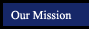CAHSEE Prep - Tutorial sources by Topic - Algebra 1
 Vocabulary Math Glossary (more sophisticated than it looks!) Finding opposites, reciprocals, and roots Finding Opposites, Reciprocals and Roots - RegentsPrep.org Solve equations and inequalities with absolute values Solving Absolute value equations - Purplemath.com Simplify expressions before solving linear equations Simplifying Linear Equations - Teacherschoice.com.au Graph linear equations Graphing Linear Equations - Regentsprep.org Verify that a point lines on a line Verify Points on a Line - Algebra-online.com Parallel Lines Parallel Lines - Regentsprep.org System of Equations Systems of Equations - Regentsprep.org Solving Problems with Algebra Solving Ratio and Proportions Problems with Algebra - Themathpage.com Data Calculations Data Calculation involving mean - Regentsprep.org Methods of Collecting, Representing, & Displaying Data Collecting, Representing and Displaying Data - Regentsprep.org Population Samples Population Samples - Mrs.umn.edu Compound Events Probability involving compound events - Regentsprep.org Independent/Dependent Events Independent/Dependent Events - Regentsprep.org

Online Scientific Calculator from Calculator.com

Math Glossary
(more sophisticated than it looks!)